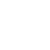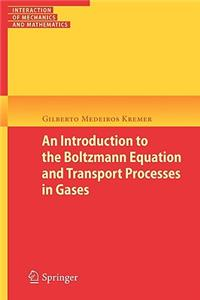Home > Technology & Engineering > Mechanical engineering & materials > Mechanical engineering > Tribology (friction & lubrication) > An Introduction to the Boltzmann Equation and Transport Processes in Gases
5%# An Introduction to the Boltzmann Equation and Transport Processes in Gases

•••••International Edition

Thisbookdealswith theclassicalkinetictheoryofgases.Itsaimisto present the basic principles of this theory within an elementary framework and from a more rigorous approach based on the Boltzmann equation. The subjects are presented in a self-contained manner such that the readers can und- stand and learn some methods used in the kinetic theory of gases in order to investigate the Boltzmann equation. In Chapter 1, a sketch on the evolution of the ideas of the kinetic theory is presented. Afterwards, the basic principles of an elementary kinetic theory areintroduced, which arebasedonthe concepts ofmean freepath, molecular mean velocity and mean free time. The Maxwellian distribution function is determinedfromstatisticalarguments, andthetransportcoe?cients ofshear viscosity, thermal conductivity and self-di?usion are obtained from the e- mentary theory. The most common interaction potentials used in the kinetic theory of gases are also introduced in this chapter, and the dynamics of a binary collision is analyzed. Chapter 2 is dedicated to the study of the Boltzmann equation. First, the BoltzmannequationisderivedandtheequationsoftheBBGKYhierarchyare determined.Fromtheknowledgeofthetransferequation-whichfollowsfrom theBoltzmannequation-themacroscopicbalanceequationsforthemoments ofthedistributionfunctionarederived.Theequilibriumdistributionfunction is determined from the Boltzmann equation and the equilibrium states of a rare?ed gas are also analyzed. In this chapter, theH-theorem and the paradoxes of Loschmidt and Zermelo are discussed. The chapter ends with an analysis of the di?erent forms of the entropy which are used in statistical mechanics to describe the canonical and microcanonical ensembles.

## Best Sellers

Product Details
• ISBN-13: 9783642116957
• Publisher: Springer
• Publisher Imprint: Springer
• Depth: 19
• Height: 234 mm
• No of Pages: 328
• Series Title: Interaction of Mechanics and Mathematics
• Weight: 462 gr
• ISBN-10: 3642116957
• Publisher Date: 29 Jun 2010
• Binding: Paperback
• Edition: 1
• Language: English
• Returnable: N
• Spine Width: 18 mm
• Width: 156 mm

## Similar Products

Write A Review
Write your own book review for An Introduction to the Boltzmann Equation and Transport Processes in Gases
•••••Top Reviews
Be the first to write a review on this book An Introduction to the Boltzmann Equation and Transport Processes in Gases